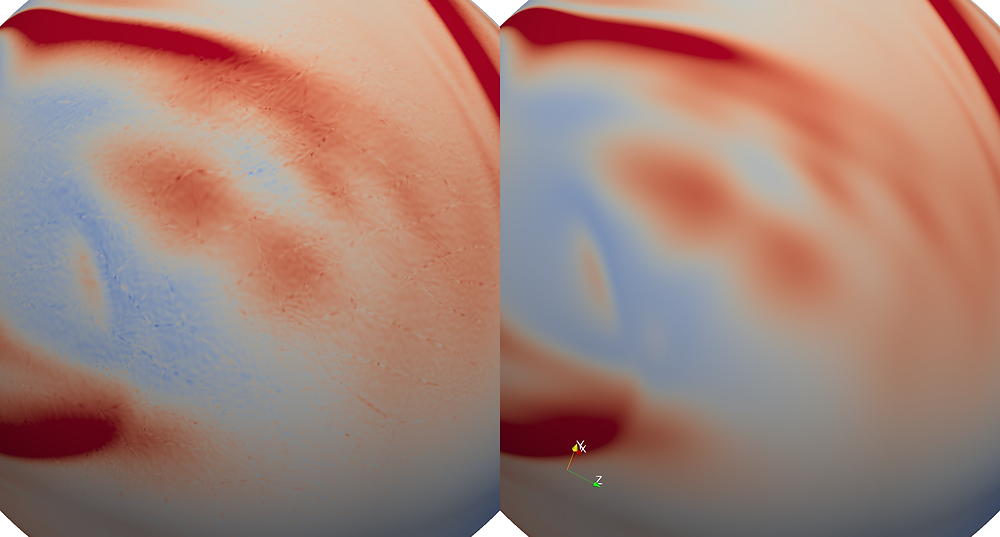# High-order Discontinuous Galerkin Simulations: is single precision enough?It is tempting to use 32 bit floating point arithmetic (FP32) on GPUs. Modern consumer grade cards from NVIDIA have theoretical peak performance of 10 TFLOPS. However, we do have to be careful about when it is safe to use single precision in high-order calculations.

The above simulation was run once with FP32 (single precision shown on the left) and run once with FP64 (double precision shown on the right). You might notice a granular nature of the vorticity field in the left single precision image. This is not an artifact of jpeg compression.

The discontinuous Galerkin discretization incorporates a term that adds jump terms scaled by a "lift parameter" to approximate derviatives in each element. The lift scaling parameter is N*(N+1)/h where h is an estimate for the edge orthogonal height of an element. In the above simulation we use high-order, degree 15 polynomials (N=15) and the mesh size h ranges from 0.1 to 0.03 which yields a lift parameter that is up to O(1e4). This means that when we add contributions from distributional derivatives of the solution jump it is quite possible that the edge contributions are subject to significant round of when using FP32 which supports up to 6 significant figures.

We visualize the disparity between FP32 (left) and FP64 (right) runs in the video below:

It is also possible that the step where we constrain the flow to the sphere s subject to round off errors. Finally, we are plotting vorticity which is a derived quantity, further amplifying any round off issues.

Take home: FP32 arithmetic may be inadequate for very high-order in DG simulations. Note that it is usually ok to use FP32 for this type of calculation at low order.